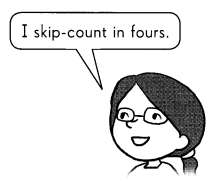# Math in Focus Grade 3 Chapter 7 Practice 1 Answer Key Mental Multiplication

Go through the Math in Focus Grade 3 Workbook Answer Key Chapter 7 Practice 1 Mental Multiplication to finish your assignments.

## Math in Focus Grade 3 Chapter 7 Practice 1 Answer Key Mental Multiplication

Multiply mentally. Fill in the missing numbers.

Example
Find 4 × 7
4 × 7 is the same as 7 × 4.
So, 4 × 7 = 28Question 1.
Find 3 × 9
3 × 9 is the same as 9 × 3.
So, 3 × 9 = ____
3 × 9 = 9 × 3 =27.

Explanation:
Given that 3 × 9 is the same as 9 × 3,
So, 3 × 9 which is 27, and 9 × 3 is also 27.

Question 2.
Find 5 × 7
5 × 7 is the same as 7 × 5.
So, 5 × 7 = ____
5 × 7 = 7 × 5 = 35.

Explanation:
Given that 5 × 7 is the same as 7 × 5,
So, 5 × 7 which is 27, and 7 × 5 is also 35.

Question 3.
Find 9 × 8
9 × 8 is the same as 8 × 9.
So, 9 × 8 = ____
9 × 8 = 8 × 9 = 72.

Explanation:
Given that 9 × 8 is the same as 8 × 9,
So, 9 × 8 which is 72, and 8 × 9 is also 72.

Multiply mentally. Fill in the missing numbers.

Example
3 × 50 = 3 × 5 tens
= 15 tens
= 150

Question 4.
3 × 500 = 3 × 5 hundreds
= ___ hundreds
= _____
3 × 500 = 1500.

Explanation:
Given that
3 × 500 = 3 × 5 hundreds
= 15 hundreds
= 1500.

Question 5.
7 × 40 = 7 × 4 tens
= ___ tens
= _____
7 × 40 = 280.

Explanation:
Given that
7 × 40 = 7 × 4 tens
= 28 tens
= 280.

Question 6.
7 × 400 = 7 × 4 hundreds
= ___ hundreds
= _____
7 × 400 = 2800.

Explanation:
Given that
7 × 400 = 7 × 4 hundreds
= 28 hundreds
= 2800.

Question 7.
8 × 60 = 8 × 6 tens
= ___ tens
= _____
8 × 60 = 480.

Explanation:
Given that
8 × 60 = 8 × 6 tens
= 48 tens
= 480.

Question 8.
8 × 600 = 8 × 6 hundreds
= ___ hundreds
= _____

Explanation:
Given that
8 × 600 = 8 × 6 hundreds
= 48 hundreds
= 4800.

Multiply mentally.

Question 9.
9 × 20 = ___
9 × 20 = 180.

Explanation:
The mental multiply of 9 × 20 is, as 9 × 2 is 18.
So 9 × 20 = 180.

Question 10.
9 × 200 = ___
9 × 200 = 1800.

Explanation:
The mental multiply of 9 × 200 is, as 9 × 2 is 18.
So 9 × 200 = 1800.

Question 11.
2 × 70 = ___
2 × 70 = 140.

Explanation:
The mental multiply of 2 × 70 is, as 2 × 7 is 14.
So 2 × 70 = 140.

Question 12.
2 × 700 = ___
2 × 700 = 1400.

Explanation:
The mental multiply of 2 × 700 is, as 2 × 7 is 14.
So 2 × 700 = 1400.

Question 13.
8 × 30 = ___
8 × 30 = 240.

Explanation:
The mental multiply of 8 × 30 is, as 8 × 3 is 24.
So 8 × 30 = 240.

Question 14.
8 × 300 = ____